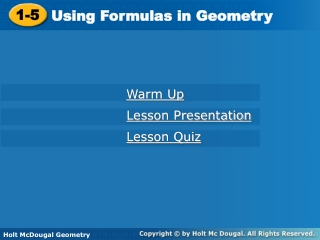DownloadDownload Presentation1-5

# 1-5

Download Presentation## 1-5

- - - - - - - - - - - - - - - - - - - - - - - - - - - E N D - - - - - - - - - - - - - - - - - - - - - - - - - - -
##### Presentation Transcript

1. 1-5 Using Formulas in Geometry Warm Up Lesson Presentation Lesson Quiz Holt McDougal Geometry Holt Geometry

2. Warm Up Evaluate. Round to the nearest hundredth. 1. 122 2. 7.62 3. 4. 5. 32() 6. (3)2 144 57.76 8 7.35 28.27 88.83

3. Objective Apply formulas for perimeter, area, and circumference.

4. Vocabulary perimeter diameter area radius base circumference height pi

5. The perimeterP of a plane figure is the sum of the side lengths of the figure. The areaA of a plane figure is the number of non-overlapping square units of a given size that exactly cover the figure.

6. The basebcan be any side of a triangle. The heighthis a segment from a vertex that forms a right angle with a line containing the base. The height may be a side of the triangle or in the interior or the exterior of the triangle.

7. Remember! Perimeter is expressed in linear units, such as inches (in.) or meters (m). Area is expressed in square units, such as square centimeters (cm2).

8. Example 1A: Finding Perimeter and Area Find the perimeter and area of each figure. = 2(6) + 2(4) = 12 + 8 = 20 in. = (6)(4) = 24 in2

9. Example 1B: Finding Perimeter and Area Find the perimeter and area of each figure. P = a + b + c = (x + 4) + 6 + 5x = 6x + 10 = 3x + 12

10. Check It Out! Example 1 Find the perimeter and area of a square with s = 3.5 in. P = 4s A = s2 P = 4(3.5) A = (3.5)2 P = 14 in. A = 12.25 in2

11. Example 2: Crafts Application The Queens Quilt block includes 12 blue triangles. The base and height of each triangle are about 4 in. Find the approximate amount of fabric used to make the 12 triangles. The area of one triangle is The total area of the 12 triangles is 12(8) = 96 in2.

12. Find the amount of fabric used to make four rectangles. Each rectangle has a length of in. and a width of in. The area of one triangle is The amount of fabric to make four rectangles is Check It Out! Example 2

13. In a circle a diameter is a segment that passes through the center of the circle and whose endpoints are on a circle. A radius of a circle is a segment whose endpoints are the center of the circle and a point on the circle. The circumference of a circle is the distance around the circle.

14. The ratio of a circle’s circumference to its diameter is the same for all circles. This ratio is represented by the Greek letter  (pi). The value of  is irrational. Pi is often approximated as 3.14 or .

15. Example 3: Finding the Circumference and Area of a Circle Find the circumference and area of a circle with radius 8 cm. Use the key on your calculator. Then round the answer to the nearest tenth.  50.3 cm  201.1 cm2

16. Check It Out! Example 3 Find the circumference and area of a circle with radius 14m.  88.0 m  615.8 m2

17. Lesson Quiz: Part I Find the area and perimeter of each figure. 1.2. 3. 23.04 cm2; 19.2 cm x2+ 4x; 4x + 8 10x; 4x + 16

18. Lesson Quiz: Part II Find the circumference and area of each circle. Leave answers in terms of . 4. radius 2 cm 5. diameter 12 ft 6. The area of a rectangle is 74.82 in2, and the length is 12.9 in. Find the width. 4² cm; 4cm2 362ft; 12ft2 5.8 in